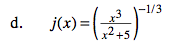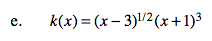### Home > CALC > Chapter 6 > Lesson 6.5.1 > Problem6-174

6-174.
1. Find the derivatives of each of the following functions. Give answers in factored form where possible. Homework Help ✎

1.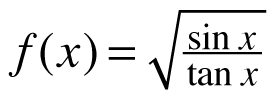2.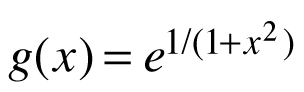3.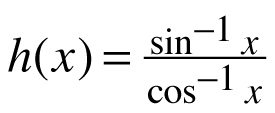4.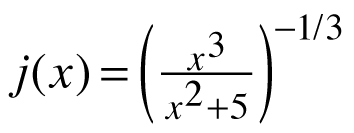5. k(x) = (x − 3)1/2 (x + 1)3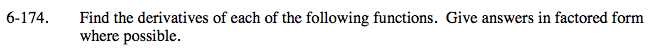To find the derivative of most of these composite functions, you will need to employ more than one shortcut: Power Rule, Chain Rule, Product Rule, Quotient Rule, and everything you know about the derivatives of trig functions and exponential functions.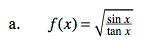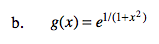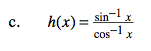Careful.
Don't confuse inverse trig with reciprocal trig functions.

sin− 1 x = arcsinx